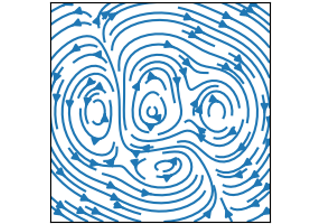# matplotlib.axes.Axes.streamplot#

Axes.streamplot(x, y, u, v, density=1, linewidth=None, color=None, cmap=None, norm=None, arrowsize=1, arrowstyle='-|>', minlength=0.1, transform=None, zorder=None, start_points=None, maxlength=4.0, integration_direction='both', broken_streamlines=True, *, data=None)[source]#

Draw streamlines of a vector flow.

Parameters:
x, y1D/2D arrays

Evenly spaced strictly increasing arrays to make a grid. If 2D, all rows of x must be equal and all columns of y must be equal; i.e., they must be as if generated by `np.meshgrid(x_1d, y_1d)`.

u, v2D arrays

x and y-velocities. The number of rows and columns must match the length of y and x, respectively.

densityfloat or (float, float)

Controls the closeness of streamlines. When `density = 1`, the domain is divided into a 30x30 grid. density linearly scales this grid. Each cell in the grid can have, at most, one traversing streamline. For different densities in each direction, use a tuple (density_x, density_y).

linewidthfloat or 2D array

The width of the streamlines. With a 2D array the line width can be varied across the grid. The array must have the same shape as u and v.

colorcolor or 2D array

The streamline color. If given an array, its values are converted to colors using cmap and norm. The array must have the same shape as u and v.

cmap, norm

Data normalization and colormapping parameters for color; only used if color is an array of floats. See `imshow` for a detailed description.

arrowsizefloat

Scaling factor for the arrow size.

arrowstylestr

Arrow style specification. See `FancyArrowPatch`.

minlengthfloat

Minimum length of streamline in axes coordinates.

start_pointsNx2 array

Coordinates of starting points for the streamlines in data coordinates (the same coordinates as the x and y arrays).

zorderint

The zorder of the streamlines and arrows. Artists with lower zorder values are drawn first.

maxlengthfloat

Maximum length of streamline in axes coordinates.

integration_direction{'forward', 'backward', 'both'}, default: 'both'

Integrate the streamline in forward, backward or both directions.

dataindexable object, optional

If given, the following parameters also accept a string `s`, which is interpreted as `data[s]` (unless this raises an exception):

x, y, u, v, start_points

broken_streamlinesboolean, default: True

If False, forces streamlines to continue until they leave the plot domain. If True, they may be terminated if they come too close to another streamline.

Returns:
StreamplotSet

Container object with attributes

This container will probably change in the future to allow changes to the colormap, alpha, etc. for both lines and arrows, but these changes should be backward compatible.

## Examples using `matplotlib.axes.Axes.streamplot`#streamplot(X, Y, U, V)

streamplot(X, Y, U, V)